# Class 8 Maths NCERT Solutions for Chapter – 3 Understanding Quadrilaterals Ex – 3.1

Question 1.
Given here are some figures :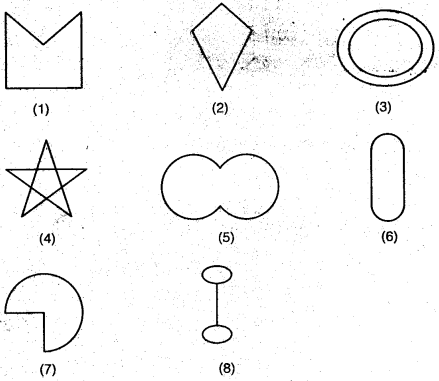Classify each of them on the basis of the following :
(a) Simple curve
(b) Simple closed curve
(c) Polygon
(d) Convex polygon
(e) Concave polygon

Solution.
The classification of the given figures is as under :
(a) Simple curve : (1), (2), (5), (6), (7) and (8)
(b) Simple closed curve : (1), (2), (5), (6) and (7)
(c) Polygon: (1) and (2)
(d) Convex polygon : (2)
(e) Concave polygon : (1) and (4)

Question 2.
How many diagonals does each of the following have?
(a)
(b) A regular hexagon
(c) A triangle.

Solution.
(a) A convex quadrilateral has two diagonals.
(b) A regular hexagon has nine diagonals.
(c) A triangle has no diagonal.

Question 3.
What is the sum of the measures of the angles of a convex quadrilateral? Will this property hold if the quadrilateral is not convex? (Make a non-convex quadrilateral and j try!)

Solution.
The sum of measures of the angles of a convex quadrilateral is 360°. Yes, this property holds in case of the quadrilateral is not convex.

Question 4.
Examine the table. (Each figure is divided into triangles and the sum of the angles deduced from that.)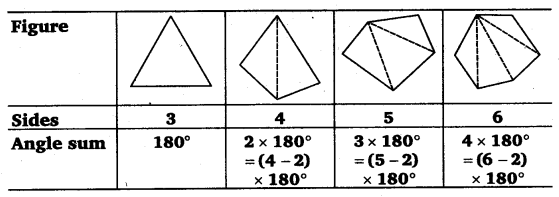What can you say about the angle sum of a convex polygon with number of sides?
(a) 7
(b) 8
(c) 10
(d) n

Solution.
From the given table, dearly we observe that the sum of angles (interior angles) of a polygon with n sides = (n – 2) x 180°.
(a) n = 7
∴ The sum of the angles of a polygon of 7 sides
Angle sum =$\left( 7-2 \right) \times { 180 }^{ \circ }$$5\times { 180 }^{ \circ }={ 900 }^{ \circ }$

(b) n = 8
∴ The sum of the angles of a polygon of 8 sides
Angle sum =$\left( 8-2 \right) \times { 180 }^{ \circ }$$6\times { 180 }^{ \circ }={ 1080 }^{ \circ }$

(c) n = 10
∴ The sum of the angles of a polygon of 10 sides
Angle sum =$\left( 10-2 \right) \times { 180 }^{ \circ }$$8\times { 180 }^{ \circ }={ 1440 }^{ \circ }$

(d) Clearly from the given table it is observed that the number of triangles is two less them the number of sides in the polygon.
∴ If the polygon has n sides, the number of triangles formed will be (n – 2).
Since the sum of angles of a triangle = 180°
∴ The sum of angles of a polygon of n sides = (n – 2) x 180°.

Question 5.
What is a regular polygon? State the name of a regular polygon of
(i)
3 slides
(ii) 4 slides
(iii) 6 slides

Solution.
A polygon is said to be a regular polygon, if all its

1. interior angles are equal;
2. sides are equal; and
3. exterior angles are euqal.

The name of a regular polygon of

1. 3 sides is equilateral triangle.
2. 4 sides is square.
3. 6 sides is regular hexagon.

Question 6.
Find the angle measure x in the following figures.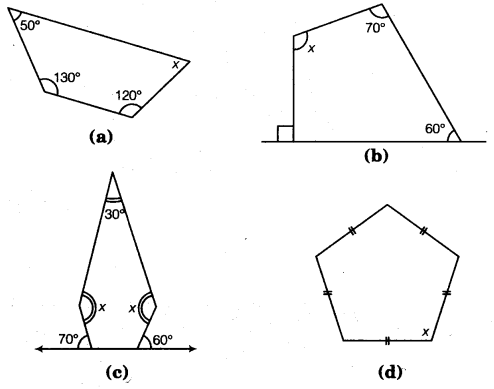Solution.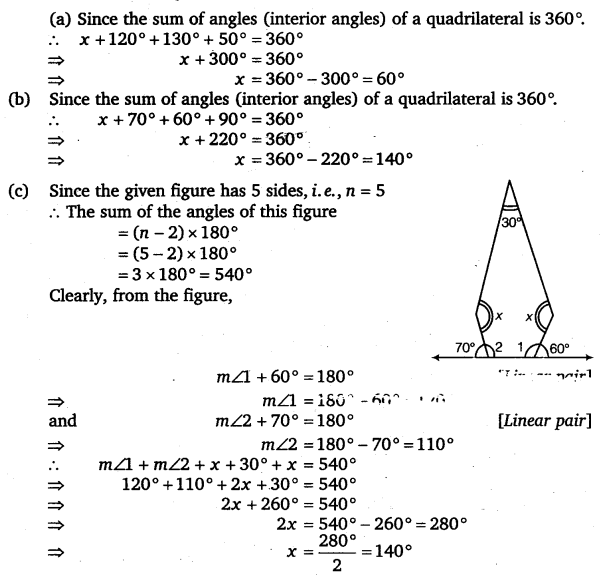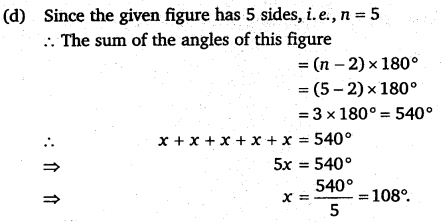Question 7.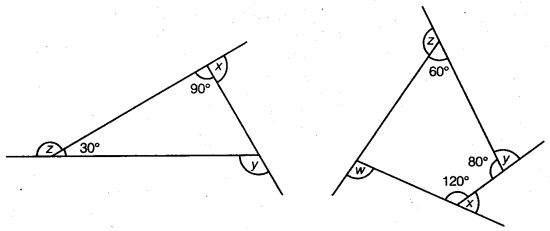(a) Find x + y + z
(b) Find x + y + z + w.

Solution.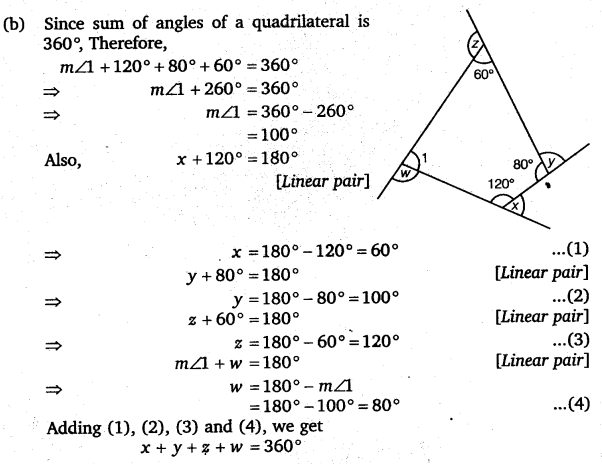### Take Your IIT JEE Coaching to Next Level with Examtube

• Mentoring & Teaching by IITians
• Regular Testing & Analysis
• Preparation for Various Engineering Entrance Exams
• Support for School/Board Exams